E58 Control Theory: Lab 5
Proportional and Integral Control of a DC Motor
in partnership with aron dobos, david luong and mark piper
March 20, 2005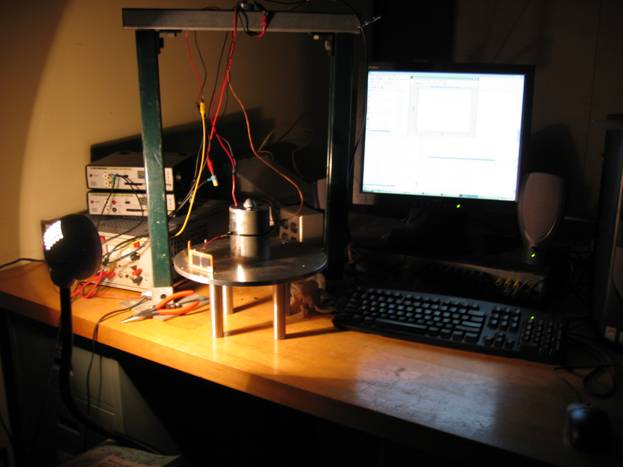abstract. PID controller video A design for a controller was created and implemented to step the angle of the satellite position as quickly as possible.  A Proportional plus Integrator plus Derivative (PID) controller was chosen for its transient and zero steady-state qualities.  A theoretical MATLAB and Simulink model was used to choose the constant parameters to obtain the quickest settling time without excessive overshoot. An extension was explored to look at dynamic PID controlling dependent on the satellite behavior during operation to find a faster response.  This is similar to real life control technique employed by NASA. satellite system. The transfer function for the satellite model used was obtained in Lab 4.  It is given below.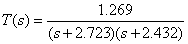This is not an exact transfer function, but seemed to model the satellite as best possible. design. We designed our controller to saturate the Kepco amplifier to obtain the quickest response.  This was done by increasing the proportional gain as well as the gain of the pure integrator. Then we increased the derivative controller slowly to decrease the overshoot.  A few iterations of this method resulted in a seemingly optimal controller that was able to step the angle of the satellite with little overshoot quickly.  A rough settling time estimate for an approximately 90 degree step was about 1 second.  The settling time criterion was within 95% of the final value. The satellite transfer function obtained in the previous laboratory exercise was not used in this design, since the ‘best’ transfer function obtained was known to have serious errors in its modeling of the system.  As a result, the experimental PID controller design methodology was used.  We did not attempt to design a phase-lead or phase-lag controller, since they necessarily would not be faster than a PID controller, and would not ensure zero steady state error.  Since the controller is for a real satellite, we do not want there to be any error. We modeled the PID controller with saturation in Simulink to compare to the experimental results, also using the lab 4 transfer function.  To ensure that our controller is stable, we plot the Nyquist diagram of the PID controller (without saturation), assuming the transfer function of the satellite is indeed that obtained in lab 4.  We chose Kp, Kd, and Ki values largely by inspection.  Having MATLAB run through the theoretical model with varying values of Kp gave us a ballpark value for a desired system response.  Similarly, this was done for Kd and Ki as well.  Shown in figures 0, step responses for these varying K values are given.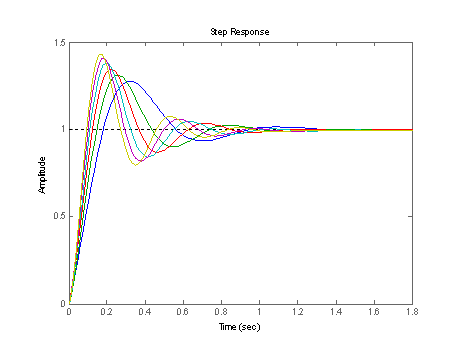Figure 0-a:  Ballparking for Kp desired values.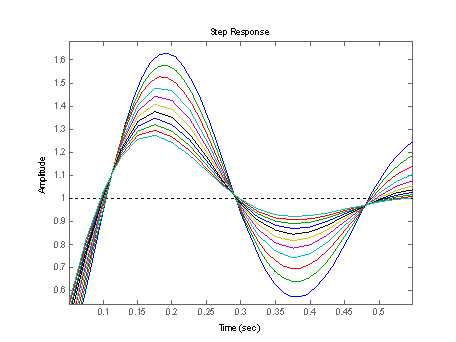Figure 0-b:  Ballparking for Kd desired values.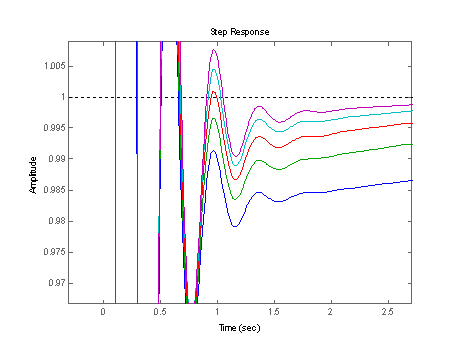Figure 0-c:  Ballparking for Ki desired values. From these graphs, we chose approximate values of Kp = 30, Kd = .5, and Ki = 20.  We tried experimentally the other constants, but these seem to give a fairly optimal response. experimental data. In Figure 2 the step response of the satellite system is shown along with the error signal and the D/A output voltage.  The angle of the satellite is assumed to be proportional to the solar cell voltage, so we denote the 'target angle' of the satellite as a voltage value.   In fact, the solar cell sensor is somewhat nonlinear, as is clear from the graph of cell voltage vs. angular displacement (Figure 1)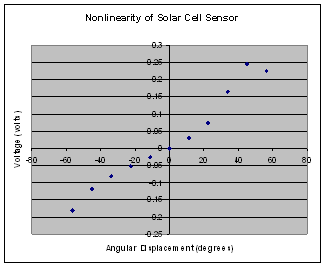Figure 1.  Nonlinearity of solar cell sensor In the cases below, a target voltage of 0.07 V (corresponding to a satellite offset of about 22 degrees) was set.  The satellite was initially at -0.15 V (-50 degrees) approximately.  Figure 2 shows clearly the PID controllers D/A voltage output.  Figure 3 shows the error signal and the actual satellite response.  The error signal clearly goes to 0, thanks to the pure integrator in the PID controller.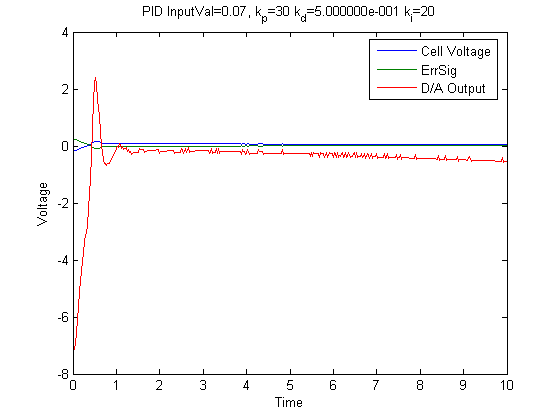Figure 2 a.  Raw Data for a 0.07 V Target Angle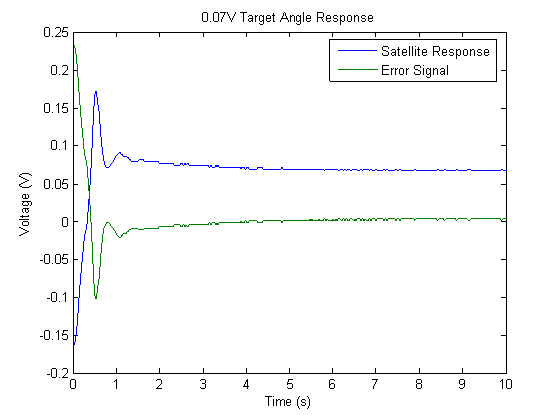Figure 2 b. Response to a desired 0.07 V Target Angle Simulink Modeling A Simulink model, shown below in figure 3 was created to look at the theoretical response of the saturating PID controller.  The saturation limits for the Simulink model were determined experimentally from the Kepco amplifier by connecting an oscilloscope in edge-triggered mode to observe the voltage being sent to the motor itself.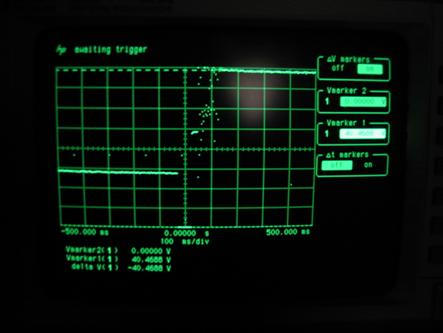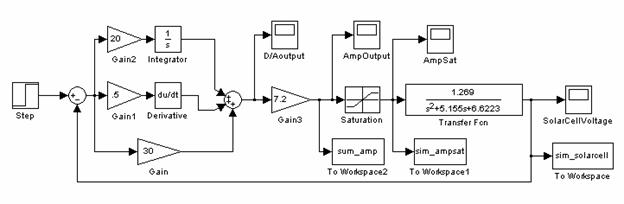Figure 3a, 3b: Measuring Saturation, Simulink Block Diagram of PID Controller   Applying a 0.23 volt step input to the system yielded the plot shown below in figure 4.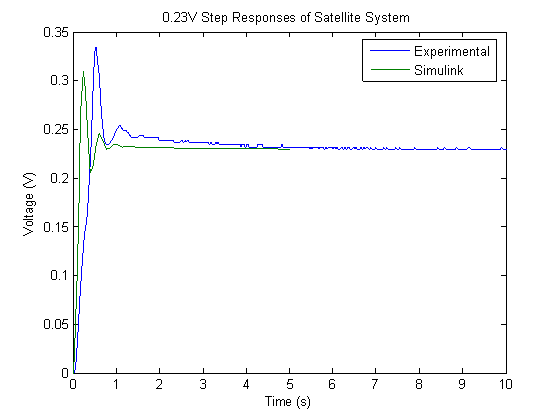Figure 4: Experimental and Theoretical Responses The non-overlap of the two curves stems largely from the fact that the transfer function of the satellite system was not accurately identified in the previous laboratory.  But the nearly identical shape of the response indicates the model was a good representation of the physical system.  stability concerns. The stability of the PID-controlled satellite system was verified using a Nyquist diagram.  The open loop gain is given below.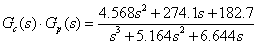The Nyquist plot is shown in Figure 5.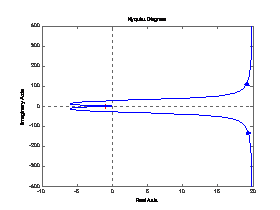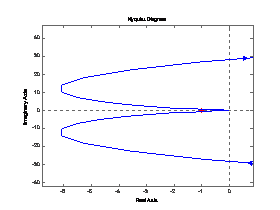Figure 5. Nyquist Stability Diagrams for the PID Controller Since the -1 point is not encircled, the system is stable, which is confirmed by our real life experiences, as the satellite did not lose control of itself. extension. As an extension, we implemented an error dependent PID controller.  In our controller, we had three ranges for the error and for every range we varied the values of Kp, Kd and Ki.  In our original PID controller, we wanted to change the Kp, Kd and Ki values, but for instance a high value for Kd at the beginning would yield bad results at the end (when the error is little).  If we had small values at the beginning then the system was slower, however with this system, we give it a kick at the beginning and decrease K at the end and this gets rid of the oscillations that we previously had.  Also with this system, we have more control overall because if the Kp, Kd and Ki values are the same for all stages, then we get the original PID system. The system can be improved by having more stages (ie four ranges of error instead of three) but that complicates the system but doesn't really add more stability or increase the speed of the system. Comparisons with the original PID design: In Figure 6 and Figure 7 you can see the responses of the original PID controller and the modified controller respectively.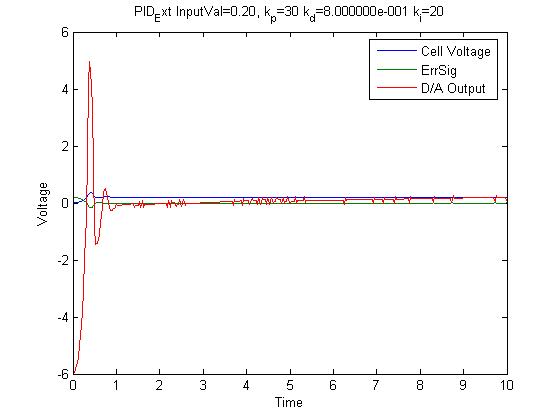Figure 6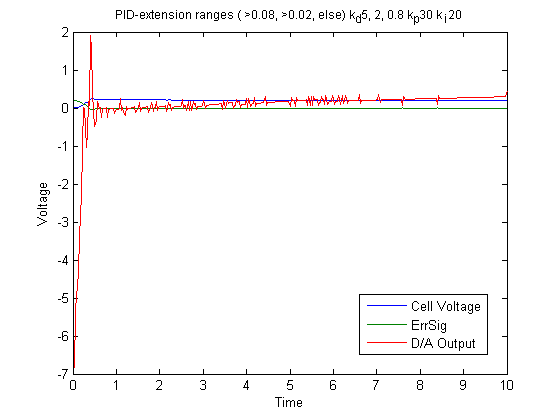Figure 7 As you can see in Figure 8 and Figure 9, the original PID controller has oscillations, higher overshoot and takes more time to settle. Clearly the modified system is better than the original controller.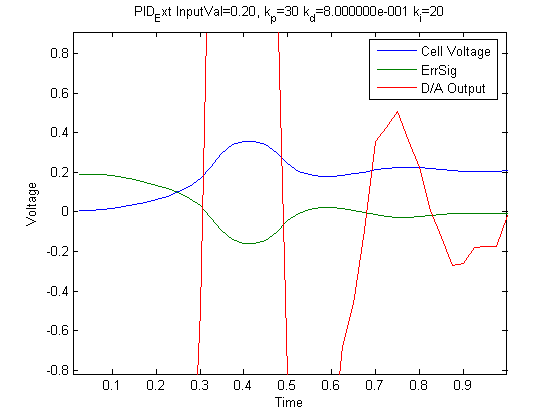Figure 8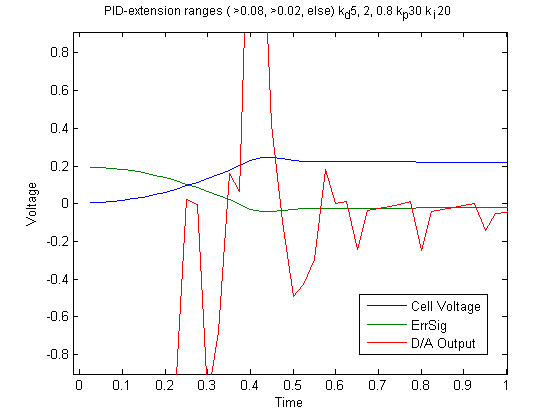Figure 9 In addition to all the benefits of the modified controller, we can also set the parameters such that the system doesn’t saturate as it did with the original controller while maintaining the same system performances.